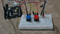Up to this point we have focused entirely on outputs, time to get our Arduino to listen, watch and feel. We’ll start with a simple pushbutton. Wiring up the pushbutton is simple. There is one component, the pull up resistor, that might seem out of place. This is included because an Arduino doesn’t sense the same way we do (ie button pressed, button unpressed). Instead it looks at the voltage on the pin and decides whether it is HIGH or LOW. The button is set up to pull the Arduino’s pin LOW when it is pressed, however, when the button is unpressed the voltage of the pin will float (causing occasional errors). To get the Arduino to reliably read the pin as HIGH when the button is unpressed, we add the pull up resistor.

### Hardware Used:

You can buy all this Hardware at Createlabz.

Arduino IDE

### Set up the Hardware:### Code:

```/*
Button

Turns on and off a light emitting diode(LED) connected to digital pin 13,
when pressing a pushbutton attached to pin 2.

The circuit:
- LED attached from pin 13 to ground
- pushbutton attached to pin 2 from +5V
- 10K resistor attached to pin 2 from ground

- Note: on most Arduinos there is already an LED on the board
attached to pin 13.

created 2005
by DojoDave <http://www.0j0.org>
modified 30 Aug 2011
by Tom Igoe

This example code is in the public domain.

http://www.arduino.cc/en/Tutorial/Button
*/

// constants won't change. They're used here to set pin numbers:
const int buttonPin = 2;     // the number of the pushbutton pin
const int ledPin =  13;      // the number of the LED pin

// variables will change:
int buttonState = 0;         // variable for reading the pushbutton status

void setup() {
// initialize the LED pin as an output:
pinMode(ledPin, OUTPUT);
// initialize the pushbutton pin as an input:
pinMode(buttonPin, INPUT);
}

void loop() {
// read the state of the pushbutton value:

// check if the pushbutton is pressed. If it is, the buttonState is HIGH:
if (buttonState == HIGH) {
// turn LED on:
digitalWrite(ledPin, HIGH);
} else {
// turn LED off:
digitalWrite(ledPin, LOW);
}
}```

### Code Breakdown:

```const int buttonPin = 2;
const int ledPin = 13;```

The difference of int and const int is that the int will change because it is a variable while the other one is a constant. We are taught by our math teachers about constant and variables. Constant are fixed no matter what you will do and Variables has an infinite number outcomes its value will depend on the equation.

```if (buttonState == HIGH) {
// turn LED on:
digitalWrite(ledPin, HIGH);
} else {
// turn LED off:
digitalWrite(ledPin, LOW);```

The if else allows greater control over the flow of code than the basic if statement, by allowing multiple tests to be grouped together. An else clause (if at all exists) will be executed if the condition in the if statement results in false. The else can proceed another if test, so that multiple, mutually exclusive tests can be run at the same time.

Each test will proceed to the next one until a true test is encountered. When a true test is found, its associated block of code is run, and the program then skips to the line following the entire if/else construction. If no test proves to be true, the default `else` block is executed, if one is present, and sets the default behavior.

Note that an else if block may be used with or without a terminating else block and vice versa. An unlimited number of such else if branches is allowed.

```pinMode(ledPin, OUTPUT);
// initialize the pushbutton pin as an input:
pinMode(buttonPin, INPUT);```

This were explained in the Blinking LED.

## Try MORE!!!

### On button off button:

The initial example may be a little underwhelming, so let’s make it a little more complicated. One button will turn the LED on and the other will the LED off. Change the code to:

```int ledPin = 13;
int buttonPin = 3;
int buttonPin2 = 2;

void setup() {
pinMpde(ledPin, OUTPUT);
pinMode(buttonPin, INPUT);
pinMode(buttonPin2, INPUT);
}
void loop() {
digitalWrite(ledPin, LOW);
}  else if (digitalRead(buttonPin2) == LOW) {
digitalWrite(ledPin, HIGH);
}```

And upload the program to your board, and start toggling the LED on and off.

Lets use the buttons to control an analog signal. To do this you will need to change the wire connecting the LED from pin 13 to pin 9, also change this code.

`int ledPin = 13; -----> int ledPin = 9;`

Add this code above the void setup() :

```const int buttonPin2 = 3;
int value = 0;```

Add this code inside the void set up():

`pinMode(buttonPin2, INPUT);`

Remove all codes from void loop() and replace with this:

```if (digitalRead(buttonPin) == LOW) { value--; }

else if (digitalRead(buttonPin2) == LOW) { value++; }
value = constrain(value, 0, 255);
analogWrite(ledPin, value);
delay(10);

}```